# Tomato Classification using Computer Vision

May. 20, 2016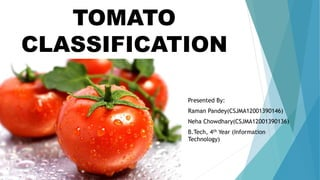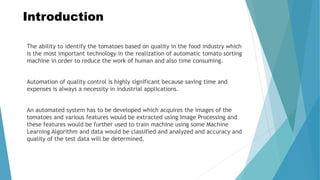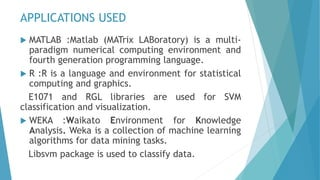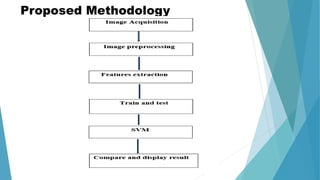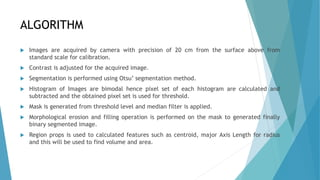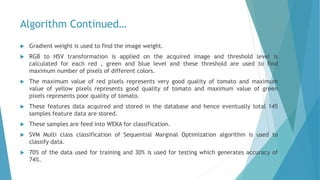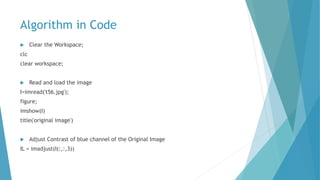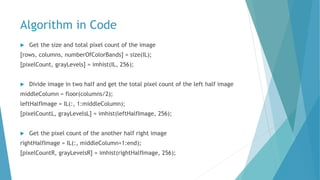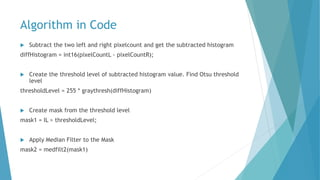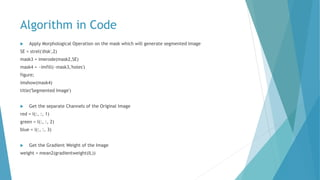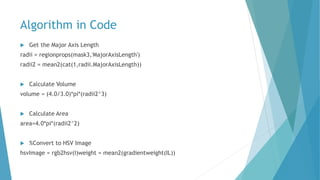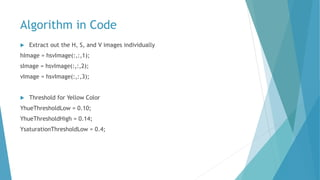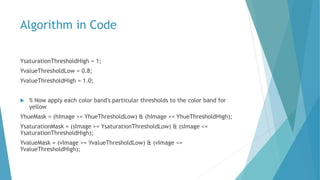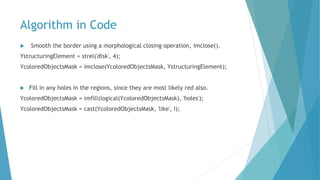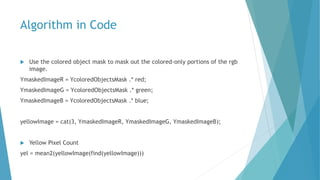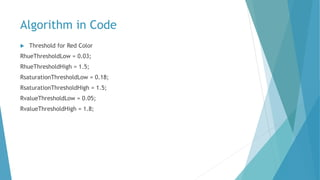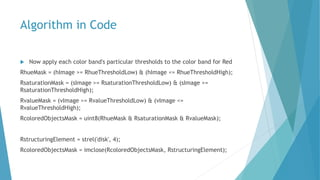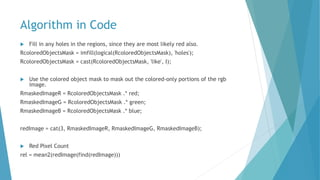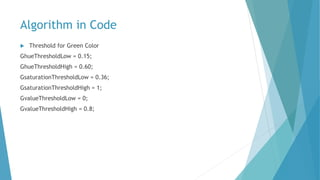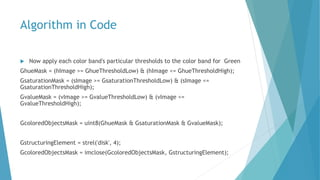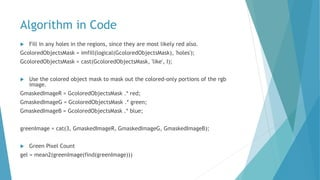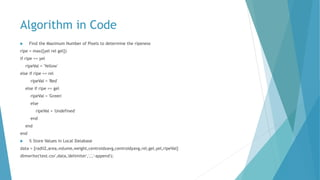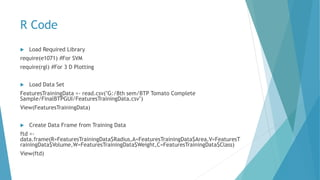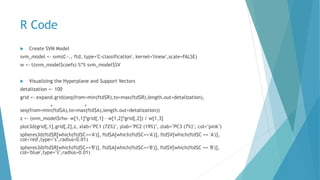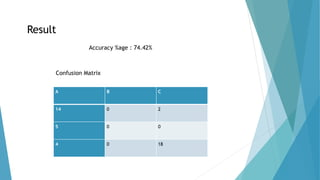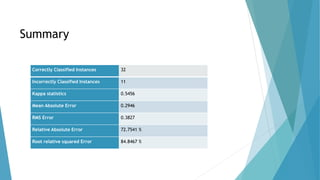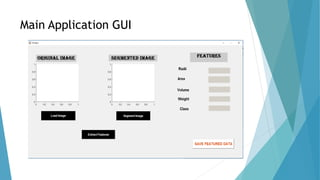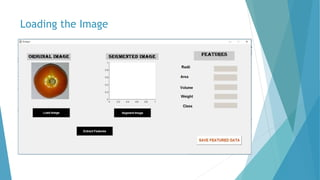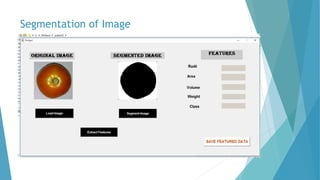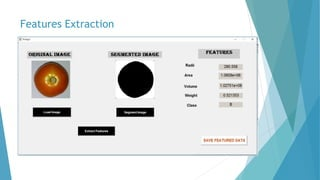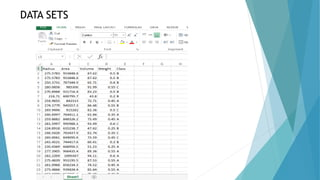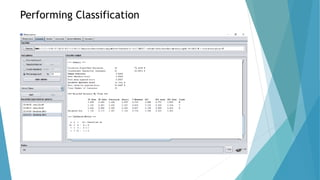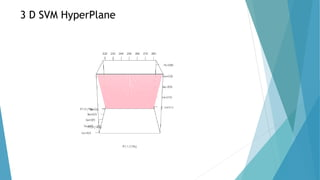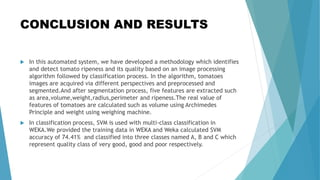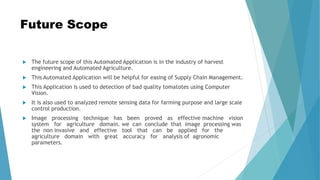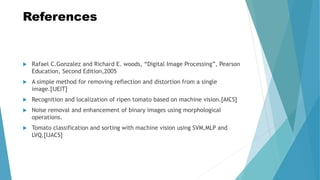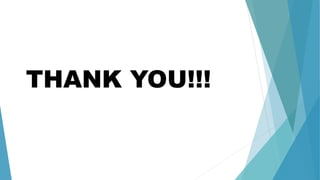1 of 38

### Tomato Classification using Computer Vision

• 1. TOMATO CLASSIFICATION Presented By: Raman Pandey(CSJMA12001390146) Neha Chowdhary(CSJMA12001390136) B.Tech, 4th Year (Information Technology)
• 2. Introduction The ability to identify the tomatoes based on quality in the food industry which is the most important technology in the realization of automatic tomato sorting machine in order to reduce the work of human and also time consuming. Automation of quality control is highly significant because saving time and expenses is always a necessity in industrial applications. An automated system has to be developed which acquires the images of the tomatoes and various features would be extracted using Image Processing and these features would be further used to train machine using some Machine Learning Algorithm and data would be classified and analyzed and accuracy and quality of the test data will be determined.
• 3. APPLICATIONS USED  MATLAB :Matlab (MATrix LABoratory) is a multi- paradigm numerical computing environment and fourth generation programming language.  R :R is a language and environment for statistical computing and graphics. E1071 and RGL libraries are used for SVM classification and visualization.  WEKA :Waikato Environment for Knowledge Analysis. Weka is a collection of machine learning algorithms for data mining tasks. Libsvm package is used to classify data.
• 5. ALGORITHM  Images are acquired by camera with precision of 20 cm from the surface above from standard scale for calibration.  Contrast is adjusted for the acquired image.  Segmentation is performed using Otsu’ segmentation method.  Histogram of Images are bimodal hence pixel set of each histogram are calculated and subtracted and the obtained pixel set is used for threshold.  Mask is generated from threshold level and median filter is applied.  Morphological erosion and filling operation is performed on the mask to generated finally binary segmented image.  Region props is used to calculated features such as centroid, major Axis Length for radius and this will be used to find volume and area.
• 6. Algorithm Continued…  Gradient weight is used to find the image weight.  RGB to HSV transformation is applied on the acquired image and threshold level is calculated for each red , green and blue level and these threshold are used to find maximum number of pixels of different colors.  The maximum value of red pixels represents very good quality of tomato and maximum value of yellow pixels represents good quality of tomato and maximum value of green pixels represents poor quality of tomato.  These features data acquired and stored in the database and hence eventually total 145 samples feature data are stored.  These samples are feed into WEKA for classification.  SVM Multi class classification of Sequential Marginal Optimization algorithm is used to classify data.  70% of the data used for training and 30% is used for testing which generates accuracy of 74%.
• 7. Algorithm in Code  Clear the Workspace; clc clear workspace;  Read and load the image I=imread('t56.jpg'); figure; imshow(I) title('original image')  Adjust Contrast of blue channel of the Original Image IL = imadjust(I(:,:,3))
• 8. Algorithm in Code  Get the size and total pixel count of the image [rows, columns, numberOfColorBands] = size(IL); [pixelCount, grayLevels] = imhist(IL, 256);  Divide image in two half and get the total pixel count of the left half image middleColumn = floor(columns/2); leftHalfImage = IL(:, 1:middleColumn); [pixelCountL, grayLevelsL] = imhist(leftHalfImage, 256);  Get the pixel count of the another half right image rightHalfImage = IL(:, middleColumn+1:end); [pixelCountR, grayLevelsR] = imhist(rightHalfImage, 256);
• 9. Algorithm in Code  Subtract the two left and right pixelcount and get the subtracted histogram diffHistogram = int16(pixelCountL - pixelCountR);  Create the threshold level of subtracted histogram value. Find Otsu threshold level thresholdLevel = 255 * graythresh(diffHistogram)  Create mask from the threshold level mask1 = IL > thresholdLevel;  Apply Median Filter to the Mask mask2 = medfilt2(mask1)
• 10. Algorithm in Code  Apply Morphological Operation on the mask which will generate segmented Image SE = strel('disk',2) mask3 = imerode(mask2,SE) mask4 = ~imfill(~mask3,'holes') figure; imshow(mask4) title('Segmented Image')  Get the separate Channels of the Original Image red = I(:, :, 1) green = I(:, :, 2) blue = I(:, :, 3)  Get the Gradient Weight of the Image weight = mean2(gradientweight(IL))
• 12. Algorithm in Code  Extract out the H, S, and V images individually hImage = hsvImage(:,:,1); sImage = hsvImage(:,:,2); vImage = hsvImage(:,:,3);  Threshold for Yellow Color YhueThresholdLow = 0.10; YhueThresholdHigh = 0.14; YsaturationThresholdLow = 0.4;
• 13. Algorithm in Code YsaturationThresholdHigh = 1; YvalueThresholdLow = 0.8; YvalueThresholdHigh = 1.0;  % Now apply each color band's particular thresholds to the color band for yellow YhueMask = (hImage >= YhueThresholdLow) & (hImage <= YhueThresholdHigh); YsaturationMask = (sImage >= YsaturationThresholdLow) & (sImage <= YsaturationThresholdHigh); YvalueMask = (vImage >= YvalueThresholdLow) & (vImage <= YvalueThresholdHigh);
• 14. Algorithm in Code  Smooth the border using a morphological closing operation, imclose(). YstructuringElement = strel('disk', 4); YcoloredObjectsMask = imclose(YcoloredObjectsMask, YstructuringElement);  Fill in any holes in the regions, since they are most likely red also. YcoloredObjectsMask = imfill(logical(YcoloredObjectsMask), 'holes'); YcoloredObjectsMask = cast(YcoloredObjectsMask, 'like', I);
• 16. Algorithm in Code  Threshold for Red Color RhueThresholdLow = 0.03; RhueThresholdHigh = 1.5; RsaturationThresholdLow = 0.18; RsaturationThresholdHigh = 1.5; RvalueThresholdLow = 0.05; RvalueThresholdHigh = 1.8;
• 17. Algorithm in Code  Now apply each color band's particular thresholds to the color band for Red RhueMask = (hImage >= RhueThresholdLow) & (hImage <= RhueThresholdHigh); RsaturationMask = (sImage >= RsaturationThresholdLow) & (sImage <= RsaturationThresholdHigh); RvalueMask = (vImage >= RvalueThresholdLow) & (vImage <= RvalueThresholdHigh); RcoloredObjectsMask = uint8(RhueMask & RsaturationMask & RvalueMask); RstructuringElement = strel('disk', 4); RcoloredObjectsMask = imclose(RcoloredObjectsMask, RstructuringElement);